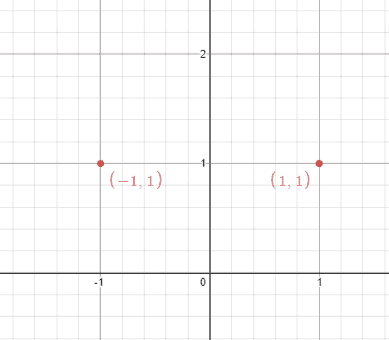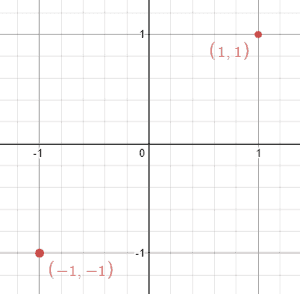# Question

Formatted question description: https://leetcode.ca/all/356.html

Given n points on a 2D plane, find if there is such a line parallel to the y-axis that reflects the given points symmetrically.

In other words, answer whether or not if there exists a line that after reflecting all points over the given line, the original points' set is the same as the reflected ones.

Note that there can be repeated points.

Example 1:Input: points = [[1,1],[-1,1]]
Output: true
Explanation: We can choose the line x = 0.


Example 2:Input: points = [[1,1],[-1,-1]]
Output: false
Explanation: We can't choose a line.


Constraints:

• n == points.length
• 1 <= n <= 104
• -108 <= points[i][j] <= 108

Follow up: Could you do better than O(n2)?

# Algorithm

The hints in the question are quite adequate, we just need to follow the hinted steps to solve the problem.

• First, we find the maximum and minimum values of the abscissa of all points, then the average of the two is the abscissa of the middle straight line,
• Then we traverse each point, and if we can find another point that is symmetrical in a straight line, we return true, otherwise we return false

# Code

• import java.nio.ByteBuffer;
import java.util.HashSet;
import java.util.Set;

public class Line_Reflection {

class Solution {
public boolean isReflected(int[][] points) {
if (points.length <= 1) {
return true;
}

int min = Integer.MAX_VALUE;
int max = Integer.MIN_VALUE;
Set<Long> pointSet = new HashSet<>();
for(int[] p: points) {
min = Math.min(min, p);
max = Math.max(max, p);
}

int sum = min + max;
for(int[] p: points) {
if(!pointSet.contains(pointToLong(sum - p, p)))
return false;
}
return true;
}

public long pointToLong(int x, int y) {
ByteBuffer bb = ByteBuffer.allocate(8);
bb.putInt(x);
bb.putInt(y);
return bb.getLong(0);
}
}

}

############

class Solution {
public boolean isReflected(int[][] points) {
int minX = Integer.MAX_VALUE, maxX = Integer.MIN_VALUE;
Set<String> pointSet = new HashSet<>();
for (int[] point : points) {
minX = Math.min(minX, point);
maxX = Math.max(maxX, point);
}
long s = minX + maxX;
for (int[] point : points) {
if (!pointSet.contains((s - point) + "." + point)) {
return false;
}
}
return true;
}
}

• class Solution:
def isReflected(self, points: List[List[int]]) -> bool:
min_x, max_x = inf, -inf
point_set = set()
for x, y in points:
min_x = min(min_x, x)
max_x = max(max_x, x)
s = min_x + max_x
for x, y in points:
if (s - x, y) not in point_set:
return False
return True

############

class Solution(object):
def isReflected(self, points):
"""
:type points: List[List[int]]
:rtype: bool
"""
if len(points) < 2:
return True
twoTimesMid = min(points) + max(points)
d = set([(i, j) for i, j in points])
for i, j in points:
if (twoTimesMid - i, j) not in d:
return False
return True